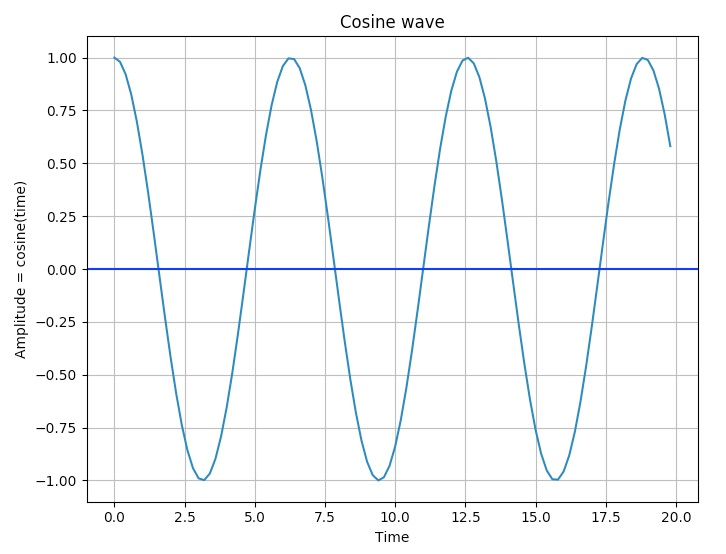# Overview:

• Cosine waves are periodic waves generated out of oscillations.

• Cosine waves are similar to sine wave however the cosine wave leads sine wave by 90 degrees of phase angle.

• The cosine curve does not go through the origin.

• The cosine wave has the same frequency as its corresponding sine wave.

• The ocean tides are an example of cosine waves.

• A cosine curve can be plotted by calling the cosine() on a numpy array and using the plot() function of the pyplot module of matplotlib.

## Example:

 import numpy as np import matplotlib.pyplot as plot   # Get x values of the cosine wave time        = np.arange(0, 20, 0.2);   # Amplitude of the cosine wave is cosine of a variable like time amplitude   = np.cos(time)   # Plot a cosine wave using time and amplitude obtained for the cosine wave plot.plot(time, amplitude)   # Give a title for the cosine wave plot plot.title('Cosine wave')   # Give x axis label for the cosine wave plot plot.xlabel('Time')   # Give y axis label for the cosine wave plot plot.ylabel('Amplitude = cosine(time)')   # Draw the grid for the graph plot.grid(True, which='both')   plot.axhline(y=0, color='b')   # Display the cosine wave plot plot.show()

## Output: